The CALCULATE function in DAX is the magic key for many calculations we can do in DAX. However, it is not pretty intuitive how it works and I spent a lot of time trying to understand how it can be used.

First of all, this is the syntax.

CALCULATE( <expression>, <filter1>, <filter2>… )

The expression that we put in the first parameter has to be evaluated to return the result (that is a value, not a table). For this reason, the expression is usually an aggregation function like SUM, MIN, MAX, COUNTROWS and so on.

This expression is evaluated in a context that is modified by the filters in the following parameters. A key point is that these filters can both enlarge and restrict the current context of evaluation. Let’s try to understand what it means by considering a few examples. The following table is the one we import in PowerPivot in a table named Demo.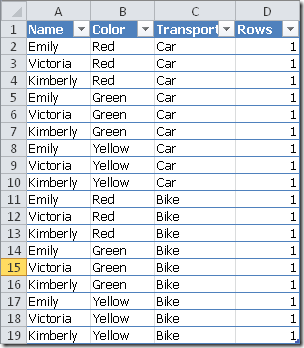If we project the count of Rows in a PivotTable putting the Name on the Rows, we get the following result: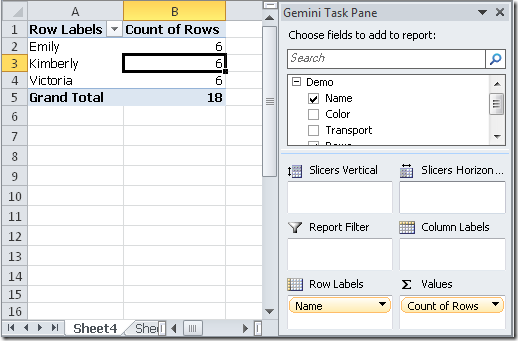Now, we might have the need to calculate a measure which is not affected by the selection of the Name or, in other words, which always calculate the context for all the names. Thus, we define this calculated measure:

CountAllNames = CALCULATE( COUNTROWS(‘Demo’),
ALL( ‘Demo'[Name] ) )

We obtain the CountAllNames column in the PivotTable that always returns the number of all the rows of the Demo table, without considering the filter on the Name.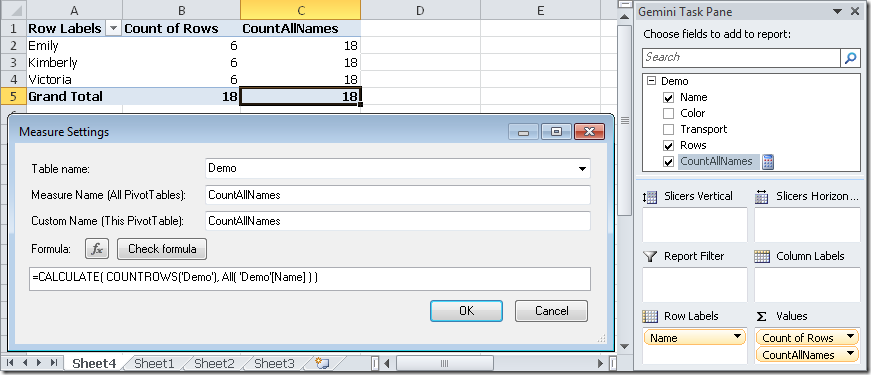However, if we add Color attribute to the rows of the PivotTable, the CountAllNames is filtered by that attribute too. In fact, for each color, we have 2 rows for a Name (see Count of Rows column) and 6 rows considering all the names (see CountAllNames column).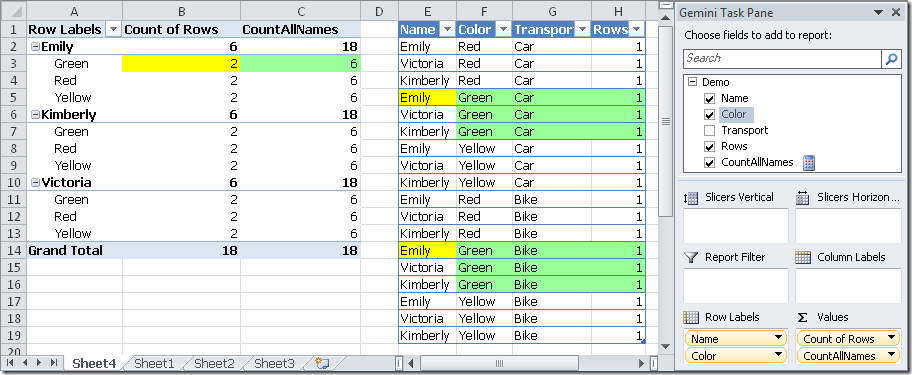Now, we can also add a Boolean expression as a filter of the CALCULATE function. For example, we might filter just the Car transport.

CountAllNamesCar = CALCULATE( COUNTROWS(‘Demo’),
ALL( ‘Demo'[Name] ),
‘Demo'[Transport] = “Car” )

In this case, we will reduce the CountAllNamesCar column for a color of Emily to 3, because the number of rows with color Green and Car transport are 3.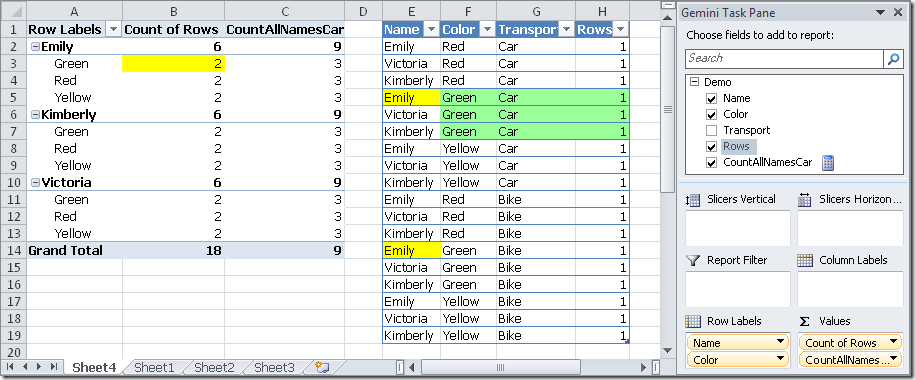At this point we might wonder whether the Calculate filter parameters are enlarging or restricting the context of evaluation. The rule is the following one.

If the current context has a filter on a column of a PowerPivot table (which is a selection of a PivotTable, regardless it is a slicer, a report filter or a row/column selection), any reference for that column in one or more filter parameters of the Calculate function replaces the existing context. Then, the filters specified in the CALCULATE parameters are combined together like they were in an AND condition of a WHERE clause of a SQL SELECT statement.

For instance, consider a filter on the Color green using a Boolean expression in the CALCULATE function:

ColorGreen = CALCULATE( COUNTROWS(‘Demo’),
‘Demo'[Color] = “Green” )

A Boolean expression used as a filter parameter in a CALCULATE function corresponds to an equivalent FILTER expression that operates on all the values of a column (for this reason, you can only a single column can be used in a Boolean expression that is used as a table filter expression):

ColorGreen = CALCULATE( COUNTROWS(‘Demo’),
FILTER( ALL(‘Demo'[Color]), ‘Demo'[Color] = “Green” ) )

We obtain that ColorGreen column always filters by color Green and each Name has only 2 rows with color Green.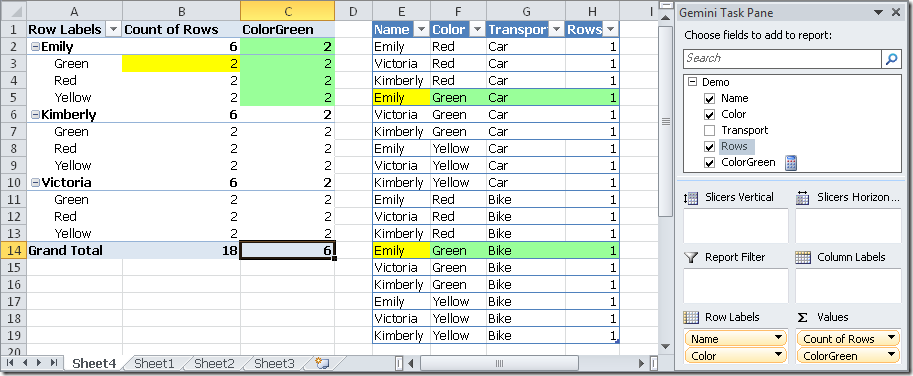Any filter expression in a CALCULATE statement overrides the existing selection of the PivotTable for the columns it contains. In the previous rule we highlighted the “reference” definition, because the FILTER that is internally used in place of the Boolean expression uses a FILTER expression that returns a set of values for the Color column. Thus, the existing selection for the color (the color is in fact specified in the rows of the PivotTable) is overridden by our filter and only green rows in the source table are considered to calculate the ColorGreen measure value. The true reason we lose the current selection on the color attribute is that the ALL( Demo[Color] ) expression returns a set of all the color values and ignores the existing selection.

If we don’t want to lose the existing selection of the PivotTable (that means that we don’t want to lose the existing filters on the calculation context), we can simply use in the FILTER expression a function that doesn’t ignore the existing selection. Instead of using the ALL( Demo[Color] ) expression as the source of the filter, we can use the VALUES( Demo[Color] ) expression, which keeps existing selections and returns the values still available in the color attribute.

In fact, if we use the following calculated measure:

ColorGreen = CALCULATE( COUNTROWS(‘Demo’),
FILTER( VALUES(‘Demo'[Color]), ‘Demo'[Color] = “Green” ) )

We obtain as a result that the Color filter in the PivotTable is still active, and returns no rows for all the colors but green.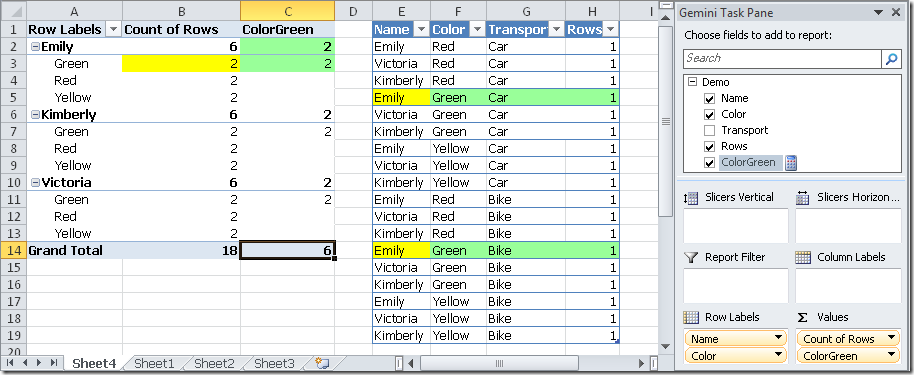We can see that the FILTER expression in a CALCULATE function always replaces the previous context for the referenced columns. However, we can save the existing context by using an expression which uses the existing context and further restricts the members we want to consider for one or more columns. And this is what we have done using VALUES formula instead of ALL as the first parameter of the FILTER call.

Thanks to Marius Dumitru, the various combination of FILTER, ALL, VALUES in a CALCULATE statement can be summarized in this way.

= CALCULATE(
COUNTROWS(‘Demo’),
FILTER( ALL(‘Demo'[Color]), ‘Demo'[Color] = “Green” ) )
— ignores/replaces existing Color filters and sets a filter on Green

= CALCULATE(
COUNTROWS(‘Demo’),
FILTER( VALUES(‘Demo'[Color]), ‘Demo'[Color] = “Green” ) )
— keeps existing Color filters and adds a further filter on Green

= CALCULATE(
COUNTROWS(‘Demo’),
FILTER( ALL(‘Demo'[Color]), ‘Demo'[Color] = “Green” ),
VALUES(‘Demo'[Color]) )
— same as the previous expression (keeps existing Color filters)
— NOTE: the first filter would consider all the colors, but the second
— expression (VALUES) only consider the current selection and
— the two filters will be considered using an AND condition, thus

= CALCULATE(
COUNTROWS(‘Demo’),
FILTER( ALL(‘Demo’), ‘Demo'[Color] = “Green” ) )
— ignores/replaces filters on all Demo columns,
— and sets a filter on all columns (Name, Transport etc., not just Color)
— with rows that meet the condition

Finally, some words of caution have to be spent to the first parameter we pass to the FILTER function. If we consider this expression:

ColorGreen = CALCULATE( COUNTROWS(‘Demo’),
FILTER( ‘Demo’, ‘Demo'[Color] = “Green” ) )

We pass the whole Demo table to the FILTER condition, which results a filter of the current context with all the columns! In this way we apply a restrictions on the color green and we get the same result as before (no rows for all the colors but green, the selection of color of the PivotTable is still applied) but, remember, the FILTER is returning ALL the rows. What does it mean?

Well, consider a further selection on the PivotTable where the Transportation attribute is filtered by Bike. This is the result using the ColorGreen definition we have just defined.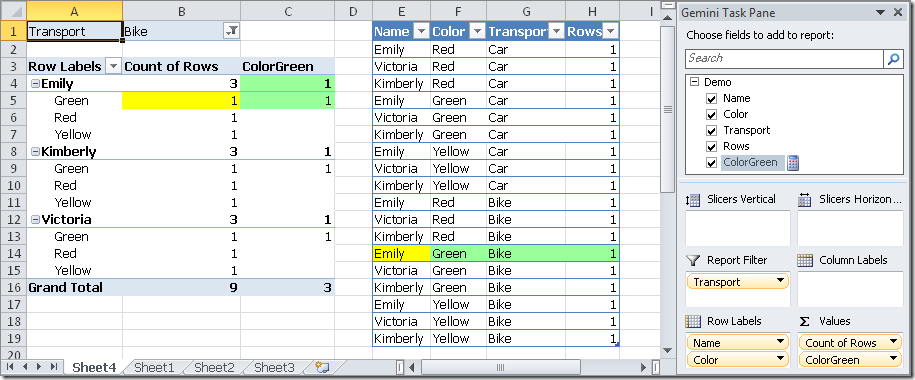Now, let’s add another filter to the CALCULATE function, filtering also the rows with Trasport equals to Car.

CarGreen = CALCULATE( COUNTROWS(‘Demo’),
FILTER( ‘Demo’, ‘Demo'[Color] = “Green”),
‘Demo'[Transport] = “Car” )

which, as we have seen before, corresponds to:

CarGreen = CALCULATE(
COUNTROWS(‘Demo’),
FILTER( ‘Demo’, ‘Demo'[Color] = “Green”),
FILTER( ALL( ‘Demo'[Transport] ), ‘Demo'[Transport] = “Car” ) )

The results is that the filter for Bike defined in the PivotTable plus the filter for Car defined in the CarGreen measure returns no rows at all in the CarGreen result. If we think about it, why this happens is not very intuitive at this point!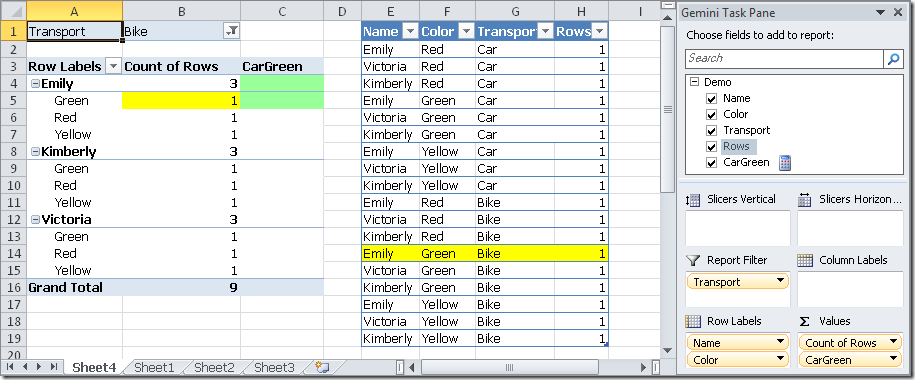Our filter on the Transport column is actually restricting the current selection and is not replacing it! But, wait, if we write just the filter on Transport, without the filter on Color, we have:

TransportCar = CALCULATE( COUNTROWS(‘Demo’),
‘Demo'[Transport] = “Car” )

that produces the following result, which replaces the Bike selection of the PivotTable in the TransportCar column!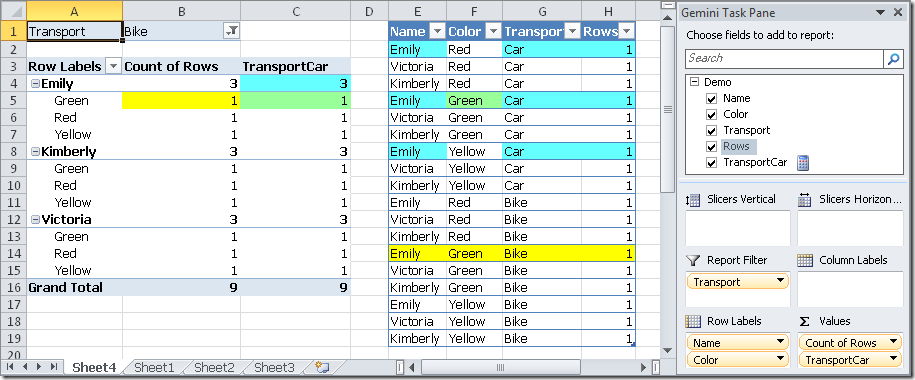This last calculation (TransportCar) is simple to explain, because it has the same behavior we have seen before with the first ColorGreen calculated measure, where the filter of the color green replaced any existing color selection in the PivotTable. The difference in the CarGreen calculation is the other filter parameter, which returns all the columns from the Demo table. Consider the highlighted filter in the CarGreen formula:

CarGreen = CALCULATE
COUNTROWS(‘Demo’),
FILTER( ‘Demo’, ‘Demo'[Color] = “Green”),
FILTER( ALL( ‘Demo'[Transport] ), ‘Demo'[Transport] = “Car” ) )

The filter on color green returns all the columns of the current context. If we consider the corresponding rows for the cell B5 of the PivotTable (Emily, Green, Bike), this is just one row (the yellow one), and this row has the Bike value for the Transport attribute. When we apply the second filter, we have a single value for the attribute Transport, which is Car. At this point, the intersection between those two sets of Transport (one is only Bike, the other is only Car) is an empty set. Thus, the result for CarGreen measure is empty, because there are no corresponding rows for the selection made.

This can be tricky, but we finally have this behavior.

• The CALCULATE function applies a calculation (the first parameter) for each cell, considering the resulting context by applying the filters (the second and following parameters) to the current context.
• Each filter can have values for one or more columns.
• Each column is computed individually in the filters expressions of the CALCULATE function
• If a column value is specified in at least one filter, it replaces the selection of the current context for that column.
• If a filter expression returns more columns, each one has its own independent set of values in the final calculation context definition
• If a column is specified in more filters, the resulting values are the intersection of these set of values (for that column).
• After all the filters have been evaluated, the intersection of the column values determines the calculation context for the expression passed as the first parameter to the CALCULATE function

Despite its complexity, this calculation is pretty fast. The key point is to understand all the side effects we have when a filter returns more columns than those we specified in the filter condition itself, which is something we have to consider carefully each time we use one or more FILTER functions inside a CALCULATE expression.

CALCULATEContext transition

Evaluates an expression in a context modified by filters.

CALCULATE ( <Expression> [, <Filter> [, <Filter> [, … ] ] ] )

SUMAdds all the numbers in a column.

SUM ( <ColumnName> )

MINReturns the smallest value in a column, or the smaller value between two scalar expressions. Ignores logical values. Strings are compared according to alphabetical order.

MIN ( <ColumnNameOrScalar1> [, <Scalar2>] )

MAXReturns the largest value in a column, or the larger value between two scalar expressions. Ignores logical values. Strings are compared according to alphabetical order.

MAX ( <ColumnNameOrScalar1> [, <Scalar2>] )

COUNTROWSCounts the number of rows in a table.

COUNTROWS ( [<Table>] )

ALLCALCULATE modifier

Returns all the rows in a table, or all the values in a column, ignoring any filters that might have been applied.

ALL ( [<TableNameOrColumnName>] [, <ColumnName> [, <ColumnName> [, … ] ] ] )

ANDChecks whether all arguments are TRUE, and returns TRUE if all arguments are TRUE.

AND ( <Logical1>, <Logical2> )

FILTERReturns a table that has been filtered.

FILTER ( <Table>, <FilterExpression> )

VALUESWhen a column name is given, returns a single column table of unique values. When a table name is given, returns a table with the same columns and all the rows of the table (including duplicates) with the additional blank row if present.

VALUES ( <TableNameOrColumnName> )